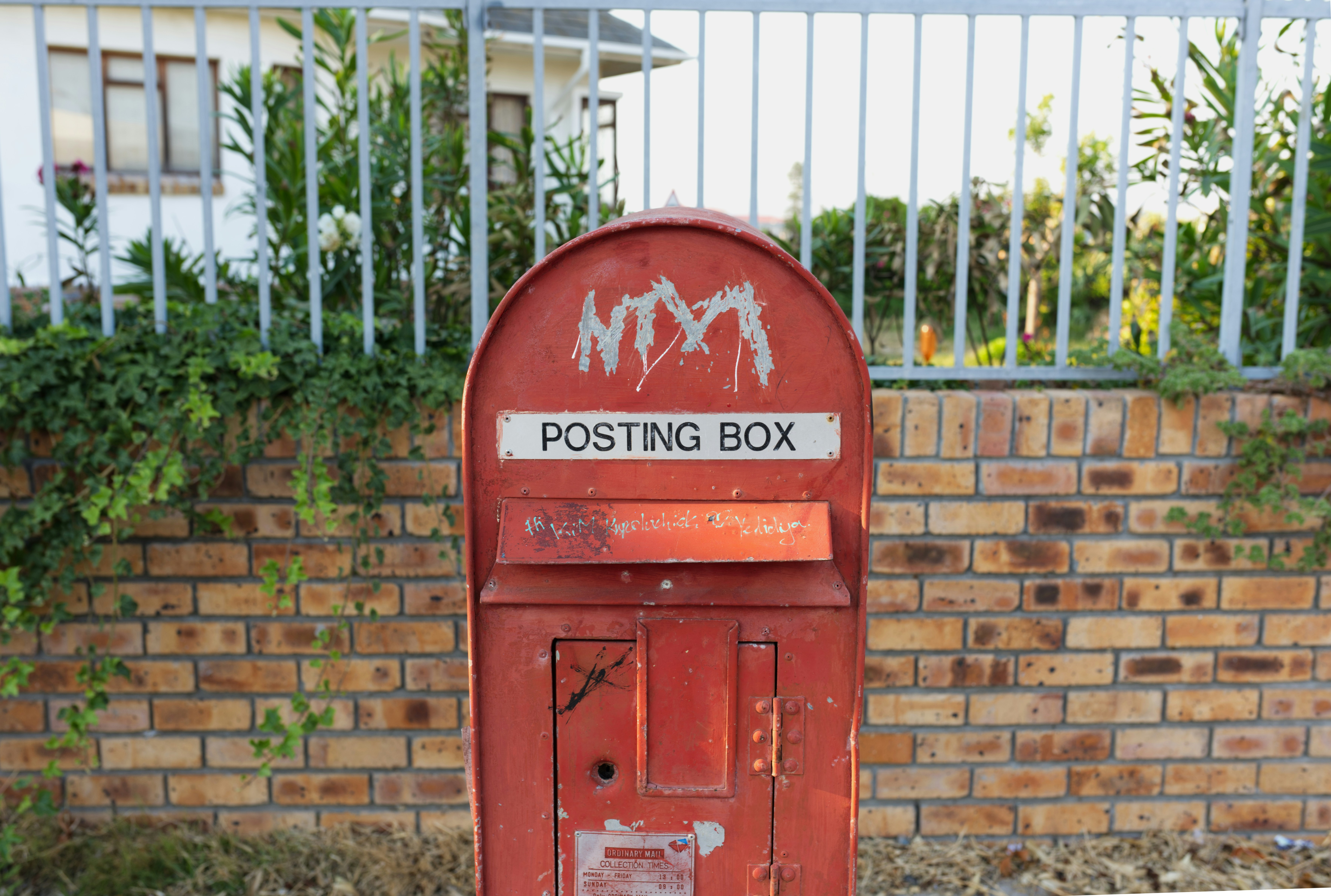Hackerrank - Validating Postal Codes Solution

# Hackerrank - Validating Postal Codes Solution

A valid postal code have to fullfil both below requirements: must be a number in the range from to inclusive. must not contain more than one alternating repetitive digit pair.A valid postal code  have to fullfil both below requirements:

1. must be a number in the range from  to  inclusive.
2. must not contain more than one alternating repetitive digit pair.

Alternating repetitive digits are digits which repeat immediately after the next digit. In other words, an alternating repetitive digit pair is formed by two equal digits that have just a single digit between them.

For example:

121426 # Here, 1 is an alternating repetitive digit.
523563 # Here, NO digit is an alternating repetitive digit.
552523 # Here, both 2 and 5 are alternating repetitive digits.

Your task is to provide two regular expressions regex_integer_in_range and regex_alternating_repetitive_digit_pair. Where:

regex_integer_in_range should match only integers range from  to  inclusive

regex_alternating_repetitive_digit_pair should find alternating repetitive digits pairs in a given string.

Both these regular expressions will be used by the provided code template to check if the input string  is a valid postal code using the following expression:

(bool(re.match(regex_integer_in_range, P))
and len(re.findall(regex_alternating_repetitive_digit_pair, P)) < 2)

Input Format

Locked stub code in the editor reads a single string denoting  from stdin and uses provided expression and your regular expressions to validate if  is a valid postal code.

Output Format

You are not responsible for printing anything to stdout. Locked stub code in the editor does that.

Sample Input 0

110000

Sample Output 0

False

Explanation 0

1 1 0000 : (0, 0) and (0, 0) are two alternating digit pairs. Hence, it is an invalid postal code.

Note:
A score of  will be awarded for using 'if' conditions in your code.
You have to pass all the testcases to get a positive score.

### Solution in Python

regex_integer_in_range = r"^[1-9]\d{5}\$"
regex_alternating_repetitive_digit_pair = r"(\d)(?=\d\1)"

import re
P = input()

print (bool(re.match(regex_integer_in_range, P))
and len(re.findall(regex_alternating_repetitive_digit_pair, P)) < 2)